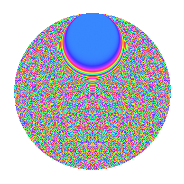# Properties

 Label 2541.2.ckLevel 2541 Weight 2 Character orbit ck Rep. character $$\chi_{2541}(19,\cdot)$$ Character field $$\Q(\zeta_{330})$$ Dimension 14080 Sturm bound 704

# Related objects

## Defining parameters

 Level: $$N$$ = $$2541 = 3 \cdot 7 \cdot 11^{2}$$ Weight: $$k$$ = $$2$$ Character orbit: $$[\chi]$$ = 2541.ck (of order $$330$$ and degree $$80$$) Character conductor: $$\operatorname{cond}(\chi)$$ = $$847$$ Character field: $$\Q(\zeta_{330})$$ Sturm bound: $$704$$

## Dimensions

The following table gives the dimensions of various subspaces of $$M_{2}(2541, [\chi])$$.

Total New Old
Modular forms 28480 14080 14400
Cusp forms 27840 14080 13760
Eisenstein series 640 0 640

## Trace form

 $$14080q + 172q^{4} - 12q^{5} + 10q^{7} + 40q^{8} - 1760q^{9} + O(q^{10})$$ $$14080q + 172q^{4} - 12q^{5} + 10q^{7} + 40q^{8} - 1760q^{9} + 66q^{10} - 20q^{11} + 48q^{14} - 12q^{15} - 192q^{16} + 60q^{17} + 10q^{18} + 36q^{22} - 20q^{23} + 90q^{24} + 180q^{25} - 24q^{26} - 30q^{28} - 40q^{29} + 18q^{31} - 18q^{33} + 80q^{35} + 344q^{36} + 70q^{37} + 24q^{38} + 90q^{40} - 6q^{42} + 104q^{44} - 12q^{45} - 70q^{46} + 12q^{47} + 2q^{49} + 20q^{51} - 198q^{52} - 132q^{53} - 24q^{56} - 6q^{58} + 12q^{60} - 30q^{61} + 10q^{63} - 176q^{64} - 36q^{66} - 64q^{67} - 180q^{68} - 138q^{70} - 10q^{72} - 90q^{73} - 40q^{74} - 24q^{78} - 94q^{79} - 180q^{80} + 1760q^{81} - 144q^{82} + 60q^{84} + 196q^{85} - 154q^{86} - 60q^{88} - 216q^{89} - 90q^{91} - 236q^{92} - 36q^{93} - 10q^{95} - 396q^{96} + O(q^{100})$$

## Decomposition of $$S_{2}^{\mathrm{new}}(2541, [\chi])$$ into newform subspaces

The newforms in this space have not yet been added to the LMFDB.

## Decomposition of $$S_{2}^{\mathrm{old}}(2541, [\chi])$$ into lower level spaces

$$S_{2}^{\mathrm{old}}(2541, [\chi]) \cong$$ $$S_{2}^{\mathrm{new}}(847, [\chi])$$$$^{\oplus 2}$$

## Hecke Characteristic Polynomials

There are no characteristic polynomials of Hecke operators in the database